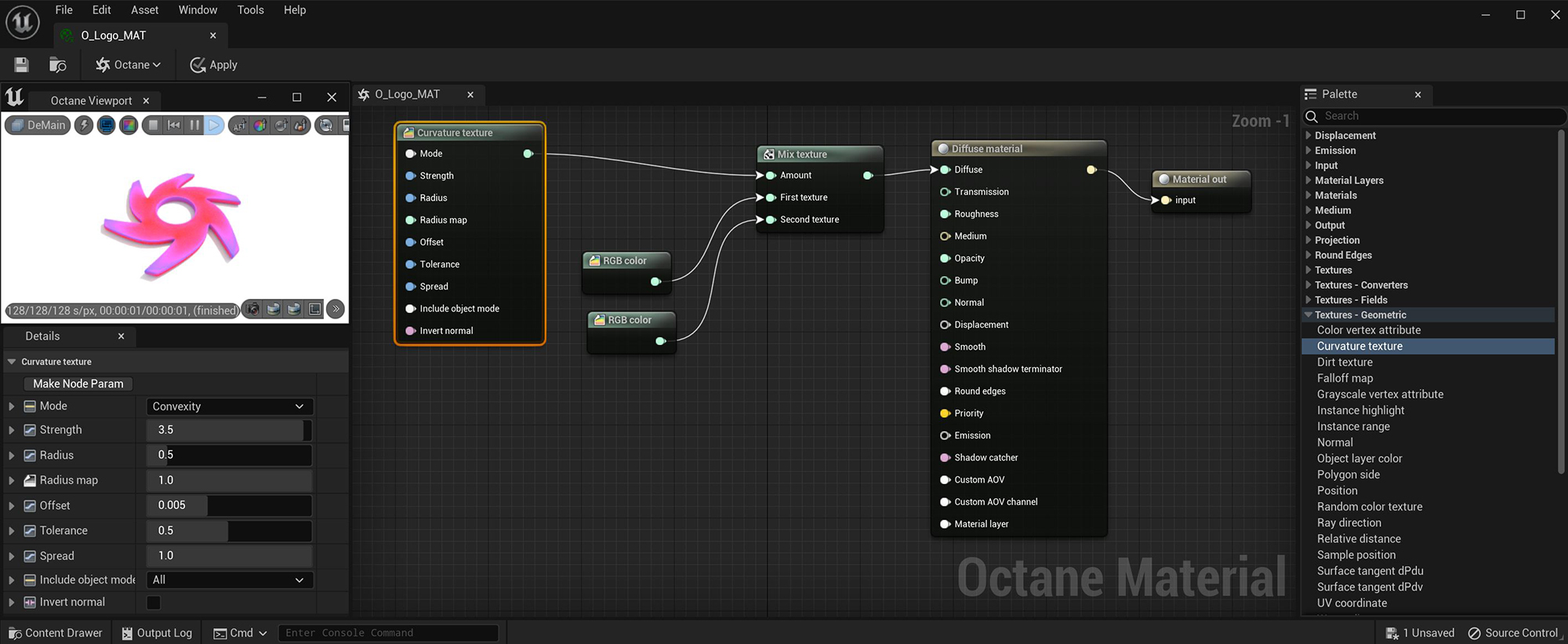# Curvature Texture

The Curvature Texture node can be used to alter the look of the edges of a surface (figure 1).Figure 1: The Curvature Texture node is used to mix Red and Blue RGB Color nodes on a Diffuse materialUsed for dull, non-reflecting materials or mesh emitters.

## Curvature Texture Parameters

Mode - Determines the type of curvature to sample.

Strength - Determines the overall strength of the curvature effect.

Radius - Specifies the maximum area affects by the curvature effect.

Radius Map- Determines the proportion of the maximum area affected by the curvature effect.

Offset - Specifies the offset from the surface used to sample the neighboring geometry.

Tolerance- Determines the tolerance for small curvature and small angles between polygons

Spread - Controls the ray direction with respect to the normal of the surface where 0 means the curvature is sampled straight in the direction of the surface normal, and 1 means the sampling rays are shot perpendicular to the surface normal.

Include Object Mode - Determines which objects should be included in calculating the curvature value.

Invert Normal - Inverts the normal direction when calculating the curvature value.